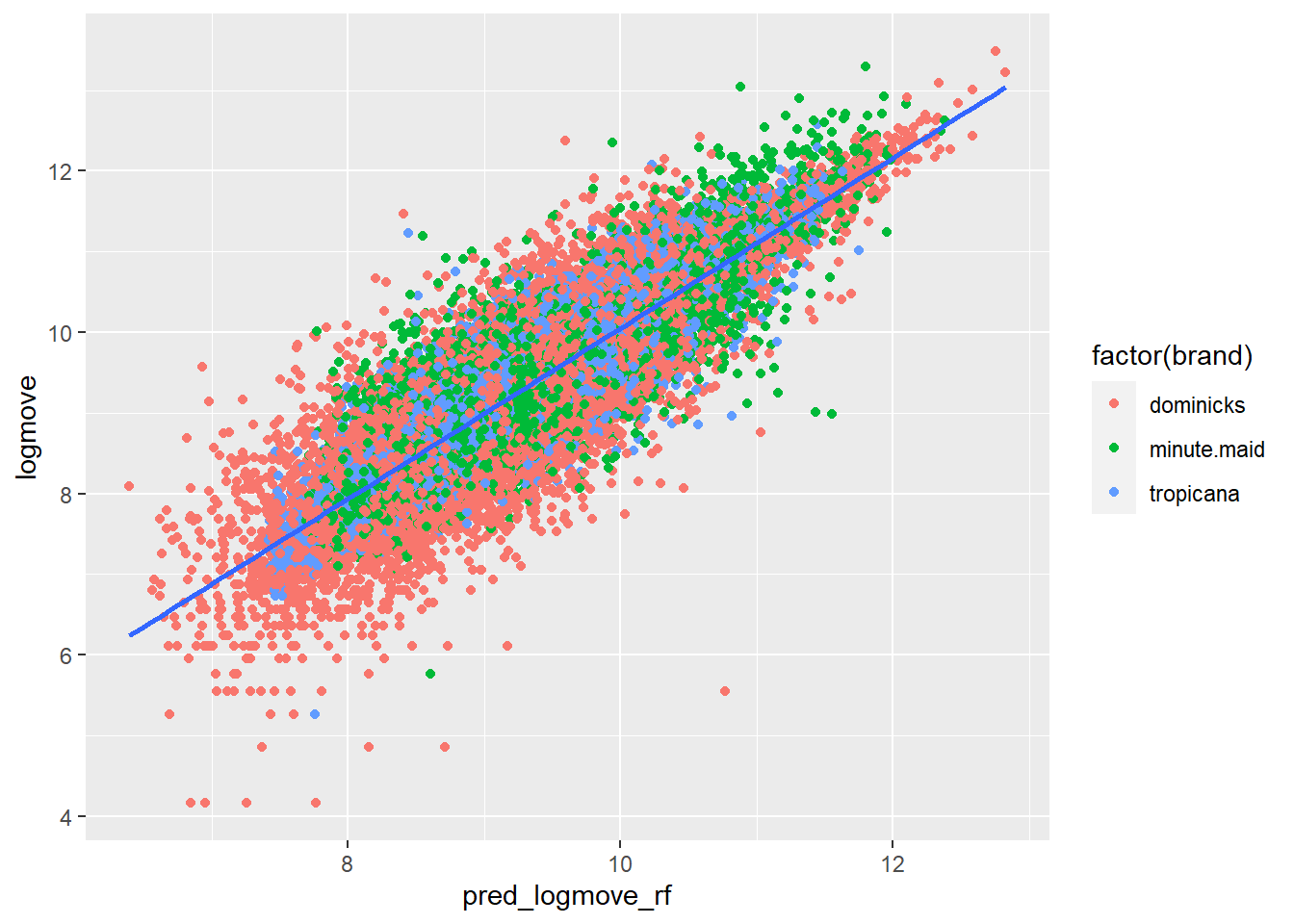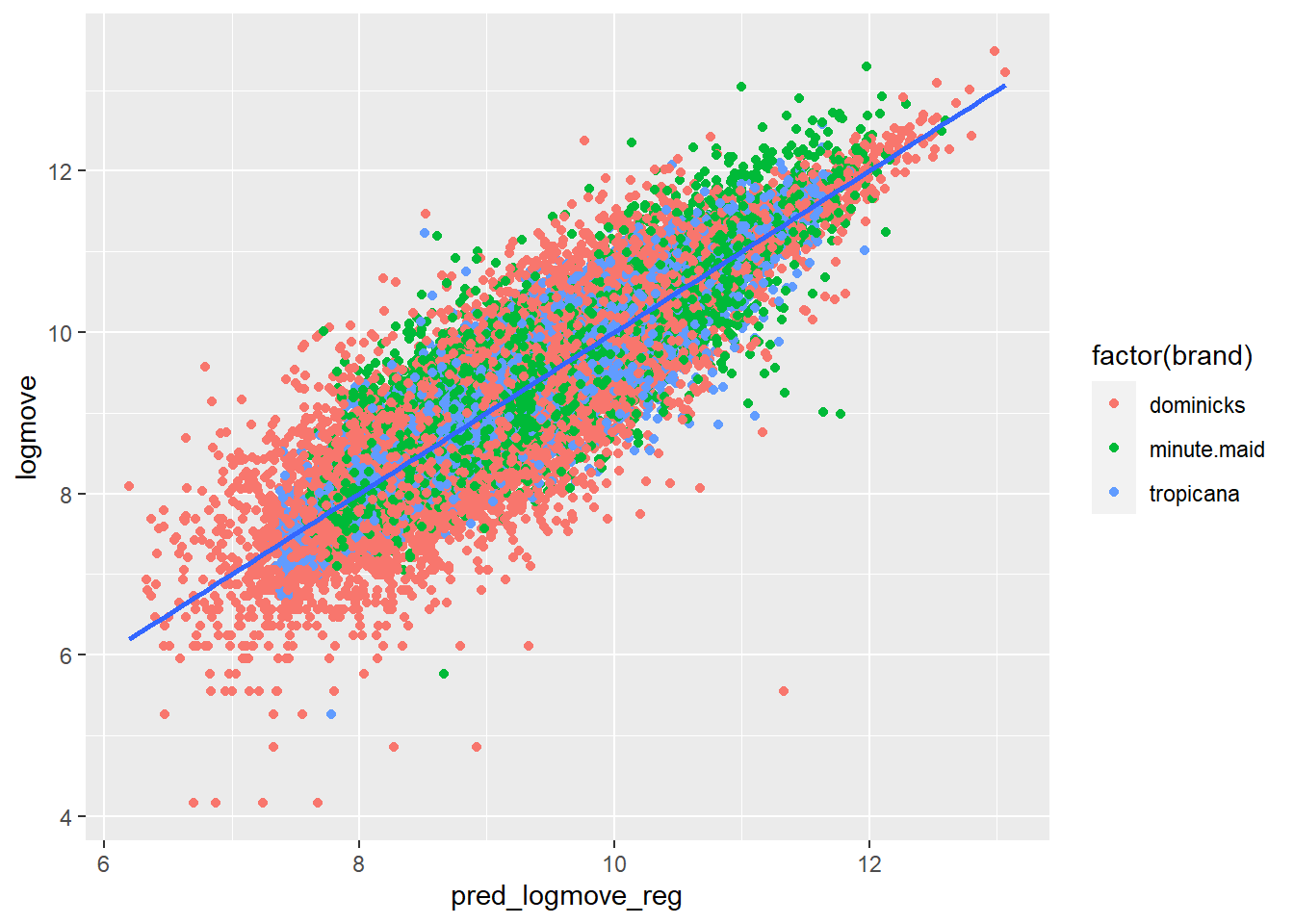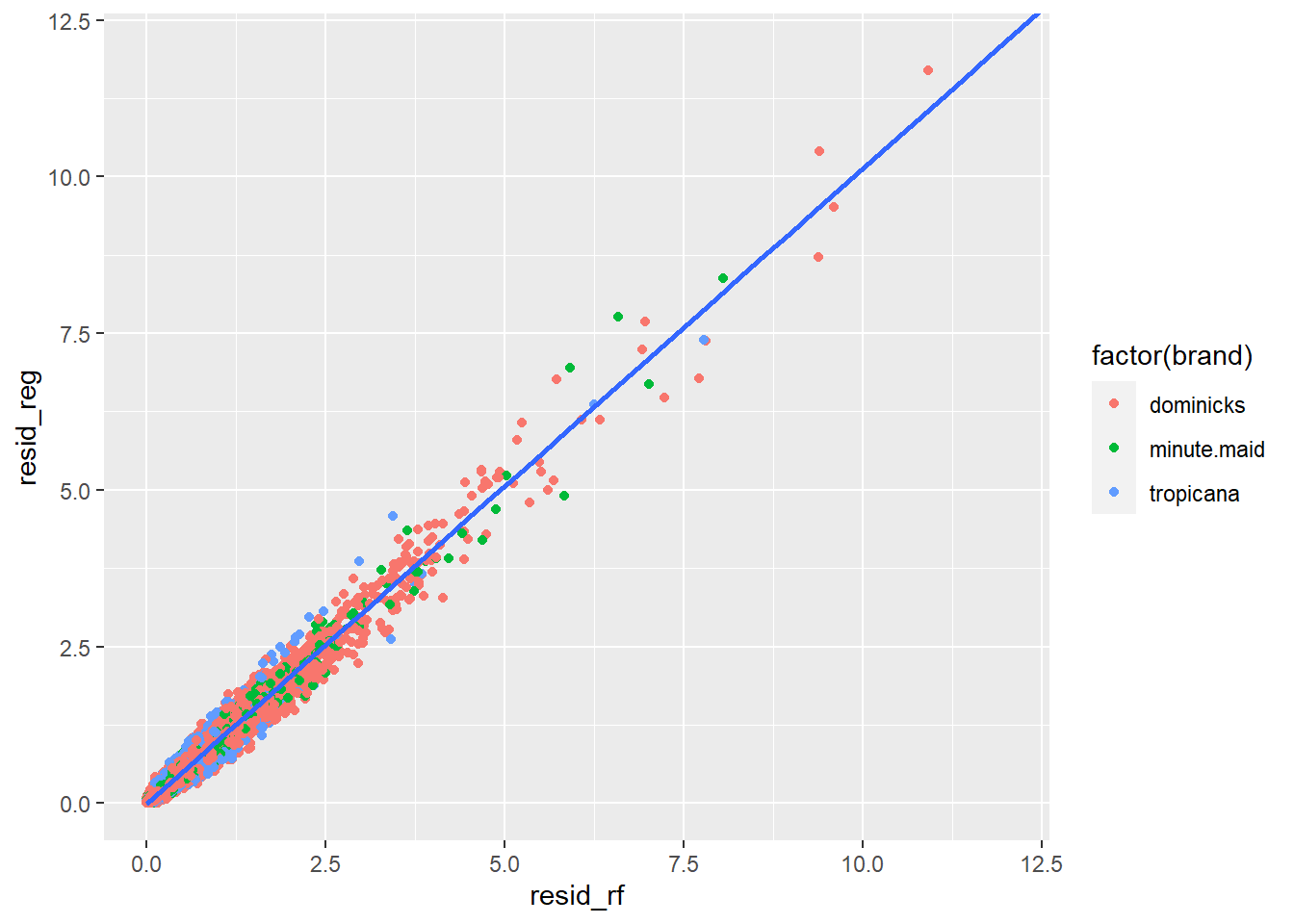## R Markdown

This is an R Markdown document. For more details on using R Markdown see http://rmarkdown.rstudio.com.

When you click the Knit button a document will be generated that includes both content as well as the output of any embedded R code chunks within the document.

The syntax on line 19 then the subsequent on line 56 tells R Markdown to run R code without the asterisks embeds the subsequent code as embedded inside the R Markdown file

library(dplyr)
##
## Attaching package: 'dplyr'
## The following objects are masked from 'package:stats':
##
##     filter, lag
## The following objects are masked from 'package:base':
##
##     intersect, setdiff, setequal, union
library(plyr)
## ------------------------------------------------------------------------------
## You have loaded plyr after dplyr - this is likely to cause problems.
## If you need functions from both plyr and dplyr, please load plyr first, then dplyr:
## library(plyr); library(dplyr)
## ------------------------------------------------------------------------------
##
## Attaching package: 'plyr'
## The following objects are masked from 'package:dplyr':
##
##     arrange, count, desc, failwith, id, mutate, rename, summarise,
##     summarize
library(doBy)
library(tidyr)
library(glmnet)
## Loading required package: Matrix
##
## Attaching package: 'Matrix'
## The following objects are masked from 'package:tidyr':
##
##     expand, pack, unpack
## Loaded glmnet 3.0-2
library(ggplot2)

library(randomForest)
## randomForest 4.6-14
## Type rfNews() to see new features/changes/bug fixes.
##
## Attaching package: 'randomForest'
## The following object is masked from 'package:ggplot2':
##
##     margin
## The following object is masked from 'package:dplyr':
##
##     combine
setwd("C:/Users/jlariv/OneDrive/Econ 404/")
data_rf <- mydata
colnames(mydata)[1-17]
##   "store"    "brand"    "week"     "logmove"  "feat"     "price"
##   "AGE60"    "EDUC"     "ETHNIC"   "INCOME"   "HHLARGE"  "WORKWOM"
##  "HVAL150"  "SSTRDIST" "SSTRVOL"  "CPWVOL5"
mydata$Q <- exp(mydata$logmove)

#reduce decimal places
is.num=sapply(mydata, is.numeric)
mydata[is.num] = lapply(mydata[is.num], round, 4)

#I'm just comparing two simple models here to highlight how cross validation and the non-linear nature of trees/forests provide better prediction.  The Figures highlight goodness of fit.

oj.rf <- randomForest(logmove ~ ., data = data_rf, ntree = 100, keep.forest = TRUE)

data_rf$pred_logmove_rf = predict(oj.rf) data_rf$resid_rf <- (data_rf$logmove - data_rf$pred_logmove_rf)^2
mean(data_rf$resid_rf) ##  0.2345915 f<-ggplot(data_rf, aes(pred_logmove_rf,logmove))+geom_point(aes(color = factor(brand))) + geom_smooth(method='lm') f ## geom_smooth() using formula 'y ~ x'reg = lm(logmove~. , data=data_rf) summary(reg) ## ## Call: ## lm(formula = logmove ~ ., data = data_rf) ## ## Residuals: ## Min 1Q Median 3Q Max ## -5.7891 -0.2531 -0.0178 0.2433 2.9534 ## ## Coefficients: ## Estimate Std. Error t value Pr(>|t|) ## (Intercept) -7.326e-01 2.564e-01 -2.857 0.00428 ** ## store -9.847e-06 9.222e-05 -0.107 0.91497 ## brandminute.maid 4.303e-02 8.461e-03 5.085 3.69e-07 *** ## brandtropicana 9.430e-02 1.258e-02 7.498 6.65e-14 *** ## week -4.928e-04 8.613e-05 -5.722 1.07e-08 *** ## feat -1.129e-01 9.109e-03 -12.394 < 2e-16 *** ## price -4.403e-02 9.450e-03 -4.659 3.19e-06 *** ## AGE60 3.457e-02 9.400e-02 0.368 0.71303 ## EDUC -2.088e-02 7.459e-02 -0.280 0.77948 ## ETHNIC -4.601e-02 2.765e-02 -1.664 0.09613 . ## INCOME -1.788e-03 2.448e-02 -0.073 0.94177 ## HHLARGE 3.044e-01 1.691e-01 1.800 0.07183 . ## WORKWOM 1.300e-01 1.075e-01 1.209 0.22675 ## HVAL150 -2.243e-03 3.072e-02 -0.073 0.94181 ## SSTRDIST 2.307e-03 1.089e-03 2.119 0.03410 * ## SSTRVOL 4.703e-03 7.189e-03 0.654 0.51298 ## CPDIST5 -7.689e-03 4.626e-03 -1.662 0.09654 . ## CPWVOL5 4.440e-02 1.951e-02 2.276 0.02288 * ## pred_logmove_rf 1.086e+00 6.148e-03 176.620 < 2e-16 *** ## resid_rf 1.497e-02 5.580e-03 2.683 0.00731 ** ## --- ## Signif. codes: 0 '***' 0.001 '**' 0.01 '*' 0.05 '.' 0.1 ' ' 1 ## ## Residual standard error: 0.4796 on 28927 degrees of freedom ## Multiple R-squared: 0.7788, Adjusted R-squared: 0.7786 ## F-statistic: 5359 on 19 and 28927 DF, p-value: < 2.2e-16 data_rf$pred_logmove_reg = predict(reg)
data_rf$resid_reg <- (data_rf$logmove - data_rf$pred_logmove_reg)^2 mean(data_rf$resid_reg)
##  0.2298855
g<-ggplot(data_rf, aes(pred_logmove_reg,logmove))+geom_point(aes(color = factor(brand))) + geom_smooth(method='lm')
g
## geom_smooth() using formula 'y ~ x'h<-ggplot(data_rf, aes(resid_rf,resid_reg))+geom_point(aes(color = factor(brand))) + coord_cartesian(xlim = c(0, 12), ylim = c(0, 12)) +
geom_smooth(method='lm')
h
## geom_smooth() using formula 'y ~ x'Note that the echo = FALSE parameter was added to the code chunk to prevent printing of the R code that generated the plot.